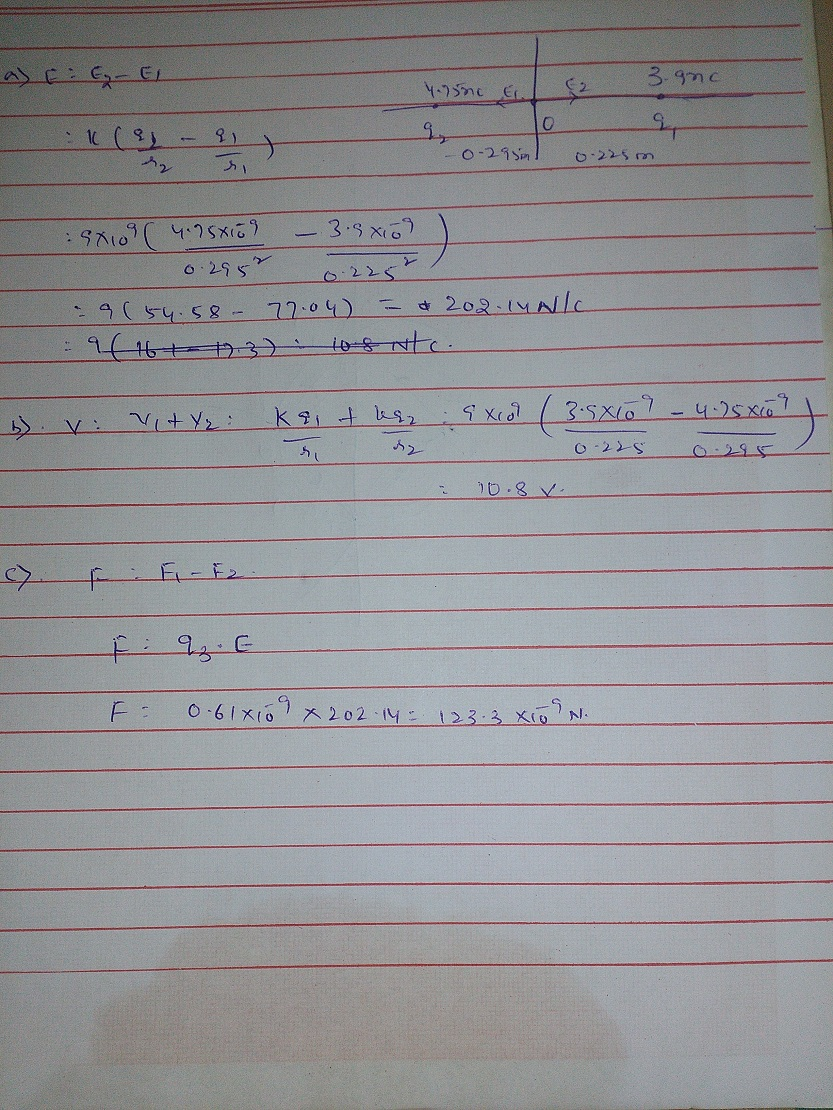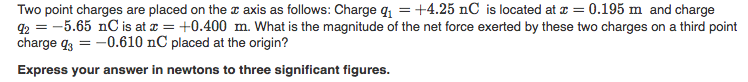Question

Two point charges are placed on the x-axis as follows: Charge q1= 3.90 nC is located...

Two point charges are placed on the x-axis as follows: Charge q1= 3.90 nC is located at x = 0.225m, and charge q2=4.75 nC is at x=-0.295 m.

(a) What are the magnitude and direction of the net electric field exerted by these two charges on the origin?

(b) What is the voltage (electrostatic potential) at the origin?

(c) What is the magnitude and direction of the electrostatic force that acts on a negative point charge q3= -0.610 nC placed at the origin?Earn Coins

Coins can be redeemed for fabulous gifts.

Similar Homework Help Questions
• Two point charges are placed on the x-axis as follows: charge q1 = 4.04 nC is...

Two point charges are placed on the x-axis as follows: charge q1 = 4.04 nC is located at x= 0.203 m , and charge q2 = 5.04 nC is at x= -0.296 m .What is the magnitude of the total force exerted by these two charges on a negative point charge q3 = -6.03 nC that is placed at the origin?

• Two point charges are placed on the z axis as follows: Charge q+4.25 nC is located...Two point charges are placed on the z axis as follows: Charge q+4.25 nC is located at 0.195 m and charge Q2 =-5.65 nC is at z = +0.400 m. What is the magnitude of the net force exerted by these two charges on a third point charge g3-0.610 nC placed at the origin? Express your answer in newtons to three significant figures. What is the direction of the net force exerted by these two charges on a negative point...

• Consider two point charges located on the x axis: one charge, q1 = -15.5 nC ,...

Consider two point charges located on the x axis: one charge, q1 = -15.5 nC , is located at x1 = -1.695 m ; the second charge, q2 = 30.5 nC , is at the origin (x=0.0000). What is the net force exerted by these two charges on a third charge q3 = 53.0 nC placed between q1 and q2 at x3 = -1.120 m ? Your answer may be positive or negative, depending on the direction of the force.

• Consider two point charges located on the x axis: one charge, q1 = -15.5 nC ,...

Consider two point charges located on the x axis: one charge, q1 = -15.5 nC , is located at x1 = -1.695 m ; the second charge, q2 = 30.5 nC , is at the origin (x=0.0000). What is the net force exerted by these two charges on a third charge q3 = 53.0 nC placed between q1 and q2 at x3 = -1.120 m ? Your answer may be positive or negative, depending on the direction of the force.

• Two point charges are located on the y-axis as follows: one charge q1 = -1.50 nC...

Two point charges are located on the y-axis as follows: one charge q1 = -1.50 nC located at y= -0.560 m, and a second charge q2 = 3.60 nC at the origin (y=0). What is the magnitude of the total force exerted by these two charges on a third charge q3 = 4.85 nC located at y3 = -0.440 m? What is the direction of the total force?

• Consider two point charges located on the x axis: one charge, q1 = -17.0 nC ,...

Consider two point charges located on the x axis: one charge, q1 = -17.0 nC , is located at x1 = -1.725 m ; the second charge, q2 = 38.0 nC , is at the origin (x=0.0000). What is the net force exerted by these two charges on a third charge q3 = 46.0 nC placed between q1 and q2 at x3 = -1.080 m ?

• Consider two point charges located on the x axis: one charge, q1 = -10.0 nC ,...

Consider two point charges located on the x axis: one charge, q1 = -10.0 nC , is located at x1 = -1.650 m ;the second charge, q2 = 36.5 nC , is at the origin (x=0.0000). What is the net force exerted by these two charges on a third charge q3 = 52.0 nC placed between q1 and q2 at x3 = -1.205 m ?

• Two point charges are placed as follows: charge q1 = -1.50 nC is at y = +6.00 m and charge q2 = +3.20 nC is at the origin. What is the total force (magnitude and direction) exerted by these two charges on a negative point charge q3 = -5.00 nC located at (

Two point charges are placed as follows: charge q1 = -1.50 nC is at y = +6.00 m and chargeq2 = +3.20 nC is at the origin. What is the total force (magnitude and direction) exerted bythese two charges on a negative point charge q3 = -5.00 nC located at (2.00 m, -4.00 m)?

• Two point charges are located on the y axis as follows: charge q1 = -2.10 nC...

Two point charges are located on the y axis as follows: charge q1 = -2.10 nC at y1 = -0.650 m , and charge q2 = 2.30 nC at the origin (y = 0). What is the magnitude of the net force exerted by these two charges on a third charge q3 = 5.50 nC located at y3 = 0.200 m ?

• Three point charges are located on the positive x-axis of a coordinate system. Charge q1 =...

Three point charges are located on the positive x-axis of a coordinate system. Charge q1 = 1.5 nC is 2.0 cm from the origin, charge q2 = -2.0 nC is 4.0 cmfrom the origin and charge q3 = 4.5 nC located at the origin. What is the magnitude of the net force exerted by the other two charges on charge q1 = 1.5 nC? What is the direction of the net force exerted by the other two charges on charge...